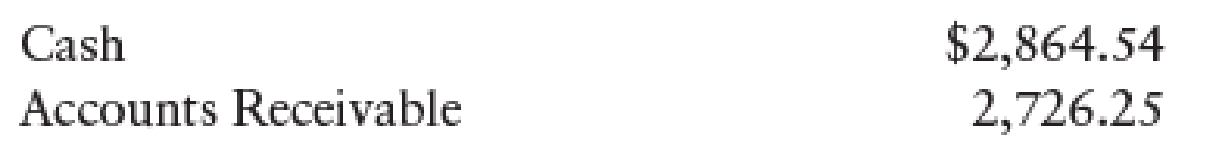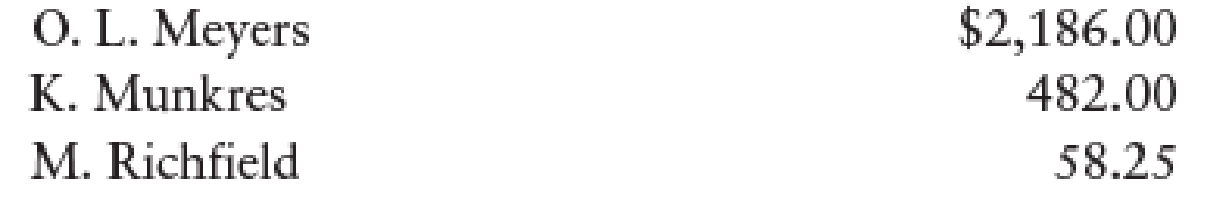Chapter 12, Problem 8SPB

Chapter
Section
Textbook Problem

SALES JOURNAL, CASH RECEIPTS JOURNAL, AND GENERAL JOURNAL Paul Jackson owns a retail business. The following sales, returns, and cash receipts are for April 20--. There is a 7% sales tax.Apr. 1    Sold merchandise to O. L. Meyers, $2,100, plus sales tax. Sale No. 111.3 Sold merchandise to Andrew Plaa,$1,000, plus sales tax. Sale No. 112.6    O. L. Meyers returned merchandise from Sale No. 111 for a credit (Credit Memo No. 42), $50, plus sales tax.7 Made cash sales for the week,$3,240, plus sales tax.9    Received payment from O. L. Meyers for Sale No. 111, less Credit Memo No. 42.12    Sold merchandise to Melissa Richfield, $980, plus sales tax. Sale No. 113.14 Made cash sales for the week,$2,180, plus sales tax.17    Melissa Richfield returned merchandise from Sale No. 113 for a credit (Credit Memo No. 43), $40, plus sales tax.19 Sold merchandise to Kelsay Munkres,$1,020, plus sales tax. Sale No. 114.21    Made cash sales for the week, $2,600, plus sales tax.24 Sold merchandise to O. L. Meyers,$920, plus sales tax. Sale No. 115.27    Sold merchandise to Andrew Plaa, $1,320, plus sales tax. Sale No. 116.28 Made cash sales for the week,$2,800, plus sales tax.Beginning general ledger account balances were as follows:Beginning customer account balances were as follows:Required 1. Record the transactions in the sales journal, cash receipts journal, and general journal. Total, verify, and rule the columns where appropriate at the end of the month. 2. Post from the journals to the general ledger and accounts receivable ledger accounts. Use account numbers as shown in the chapter.

1.

To determine

Prepare the transaction in the sales journal, cash receipts journal and general journal and verify the total column and rule the column.

Explanation

Sales Journal

Sales journal is one form of special journal book, which records all the sales transactions that are sold to customers on credit. In a single column sales journal, debit aspect of accounts receivable and credit aspect of inventory are recorded, and then posted to individual subsidiary customer account.

Prepare the given transaction in a sales journal and verify the total column and rule the column:

Table (1)

Working note 1:

Calculate the amount of accounts receivable (debit) on dated 1st April:

Accounts receivable (debit) on dated 1stApril}=(Sales + 7% on sales tax)=[$2,100+(7%×$2,100)]=[$2,100+$147]=$2,247 Working note 2: Calculate the amount of accounts receivable (debit) on dated 3rd April: Accounts receivable (debit) on dated 3rdApril}=(Sales + 7% on sales tax)=[$1,000+(7%×$1,000)]=[$1,000+$70]=$1,070

Working note 3:

Calculate the amount of accounts receivable (debit) on dated 12th April:

Accounts receivable (debit) on dated 12thApril}=(Sales + 7% on sales tax)=[$980+(7%×$980)]=[$980+$68.6]=$1,048.60 Working note 4: Calculate the amount of accounts receivable (debit) on dated 19th April: Accounts receivable (debit) on dated 19thApril}=(Sales + 7% on sales tax)=[$1,020+(7%×$1,020)]=[$1,020+$71.4]=$1,091.40

Working note 5:

Calculate the amount of accounts receivable (debit) on dated 24th April:

Accounts receivable (debit) on dated 24thApril}=(Sales + 7% on sales tax)=[$920+(7%×$920)]=[$920+$64.4]=$984.40 Working note 6: Calculate the amount of accounts receivable (debit) on dated 27th April: Accounts receivable (debit) on dated 27thApril}=(Sales + 7% on sales tax)=[$1,320+(7%×$1,320)]=[$1,320+$92.4]=$1,412.40

Verification of total debit and credit column:

Total Debit = Total Credit$7,853.80=($7,340.00+$513.80) Cash Receipts Journal: It is a special book where only cash receipts transactions that are received from customers, merchandise sales and service made in cash and collection of accounts receivable are recorded. The following are the some examples of transactions that would be recorded in the Other Accounts credit column of the cash receipts journal: • • Cash received as interest on notes payable • • Interest revenue received from debtors • • Cash receipts from bank loans • • Cash receipts for capital investments Prepare the given transactions in the cash receipts journal and verify the total column and rule the column: Table (2) Working note 1: Calculate the amount of cash on dated 7th April: Cash(debit)on dated 7th April}=(Cash sales + 7% on sales tax)=[$3,240+(7%×$3,240)]=[$3,240+\$226

2.

To determine

Post the journals to the general ledger, and the accounts receivable ledger accounts.

Still sussing out bartleby?

Check out a sample textbook solution.

See a sample solution

The Solution to Your Study Problems

Bartleby provides explanations to thousands of textbook problems written by our experts, many with advanced degrees!

Get Started

DISCOUNTED PAYBACK Refer to problem 11-1. What is the projects discounted payback?

Fundamentals of Financial Management, Concise Edition (MindTap Course List)

What are the advantages of a partnership?

Foundations of Business (MindTap Course List)

List the four components of GDP. Give an example of each.

Principles of Macroeconomics (MindTap Course List)

FINANCING ALTERNATIVES The Severn Company plans to raise a net amount of 270 million to finance new equipment i...

Fundamentals of Financial Management, Concise Edition (with Thomson ONE - Business School Edition, 1 term (6 months) Printed Access Card) (MindTap Course List)